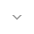# CircularArray

``` public final class CircularArray ```
``` extends Object ``` ``` ```

 java.lang.Object ↳ android.support.v4.util.CircularArray

CircularArray is a generic circular array data structure that provides O(1) random read, O(1) prepend and O(1) append. The CircularArray automatically grows its capacity when number of added items is over its capacity.

## Summary

### Public constructors

``` CircularArray() ```

Creates a circular array with default capacity.

``` CircularArray(int minCapacity) ```

Creates a circular array with capacity for at least `minCapacity` elements.

### Public methods

``` void``` ``` addFirst(E e) ```

Add an element in front of the CircularArray.

``` void``` ``` addLast(E e) ```

Add an element at end of the CircularArray.

``` void``` ``` clear() ```

Remove all elements from the CircularArray.

``` E``` ``` get(int n) ```

Get nth (0 <= n <= size()-1) element of the CircularArray.

``` E``` ``` getFirst() ```

Get first element of the CircularArray.

``` E``` ``` getLast() ```

Get last element of the CircularArray.

``` boolean``` ``` isEmpty() ```

Return true if size() is 0.

``` E``` ``` popFirst() ```

Remove first element from front of the CircularArray and return it.

``` E``` ``` popLast() ```

Remove last element from end of the CircularArray and return it.

``` void``` ``` removeFromEnd(int numOfElements) ```

Remove multiple elements from end of the CircularArray, ignore when numOfElements is less than or equals to 0.

``` void``` ``` removeFromStart(int numOfElements) ```

Remove multiple elements from front of the CircularArray, ignore when numOfElements is less than or equals to 0.

``` int``` ``` size() ```

Get number of elements in the CircularArray.

### Inherited methodsFrom class ``` java.lang.Object ```

## Public constructors

### CircularArray

`CircularArray ()`

Creates a circular array with default capacity.

### CircularArray

`CircularArray (int minCapacity)`

Creates a circular array with capacity for at least `minCapacity` elements.

Parameters
`minCapacity` `int`: the minimum capacity, between 1 and 2^30 inclusive

## Public methods

`void addFirst (E e)`
`e`